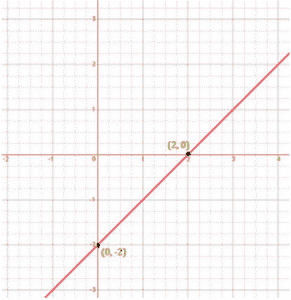# Draw the graph of the following linear equations in two variables: x-y = 2

To draw the graph x–y = 2, we have to find the values which x and y can have, satisfying the equation.

We can check that when, x = 0, y = -2,

When x = 0,

x–y = 2

0 – y = 2

y = – 2

When x = 2,

x–y = 2

2–y = 2

– y = 2–2

–y = 0

y = 0

are solutions of the given equation. So, we can use the following table to draw the graph:

 x y 0 -2 2 0

The points to be plotted are (0, – 2) and (2, 0)